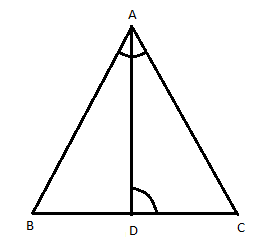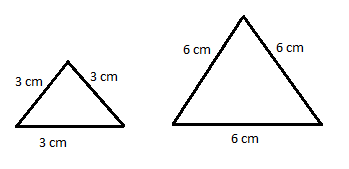Courses
Courses for Kids
Free study material
Offline Centres
MoreLast updated date: 02nd Dec 2023
Total views: 381.9k
Views today: 11.81k

# D is a point on the side of BC of a triangle ABC such that $\angle ADC=\angle BAC$. Show that $C{{A}^{2}}=CB.CD$.Verified
381.9k+ views
Hint: Draw the rough figure. Consider $\Delta ABC$ and$\Delta ADC$. Prove they are similar by AA criteria. Using AA similarity, postulate if 2 triangles are similar then corresponding sides are proportional. Hence equate sides.

Let us draw a rough figure of triangle ABC such that D is a point of the side BC (i.e. base) of the triangle. Then join AD. It is given that $\angle ADC$ is equal to $\angle BAC$ which is given in the figure, i.e. $\angle ADC=\angle BAC$.We need to prove that $C{{A}^{2}}=CB.CD$.

This can be rearranged and written as,
\begin{align} & CA.CA=CB.CD \\ & \Rightarrow \dfrac{CA}{CD}=\dfrac{CB}{CA} \\ \end{align}

Now let us consider $\Delta ABC$and$\Delta ADC$.
$\angle ACB=\angle ACD$, they are common angles of both the triangles and it is given that$\angle ADC=\angle BAC$.

Thus by AA similarity criterion we can say that the two triangles are similar.

Here in the AA criterion, two triangles are similar if all the corresponding angles are congruent and their corresponding sides will be proportional, i.e. by AA similarity postulate, if two angles in one triangle are congruent to two angles in another triangle, then the two triangles are similar.

$\therefore \Delta BAC\tilde{\ }\Delta ADC$.
We said that if two triangles are equal then their corresponding sides are proportional, which means we get,
$\dfrac{BA}{AD}=\dfrac{AC}{CD}=\dfrac{BC}{AC}$

Hence if we are taking, $\dfrac{AC}{CD}=\dfrac{BC}{AC}$, i.e. $\dfrac{CA}{CD}=\dfrac{CB}{CA}$.

We get by cross multiplying,
\begin{align} & CA.CA=CB.CD \\ & \Rightarrow C{{A}^{2}}=CB.CD \\ \end{align}

Hence we proved what was asked in the question.

Note: The mathematical definition for similar triangles states that the triangles having proportional sides and all corresponding angles are the same.If we consider there are two triangles, where each side of the larger triangle is twice as large as the smaller triangle, then by AA criteria, the triangles are similar if two corresponding angles are similar to each other.